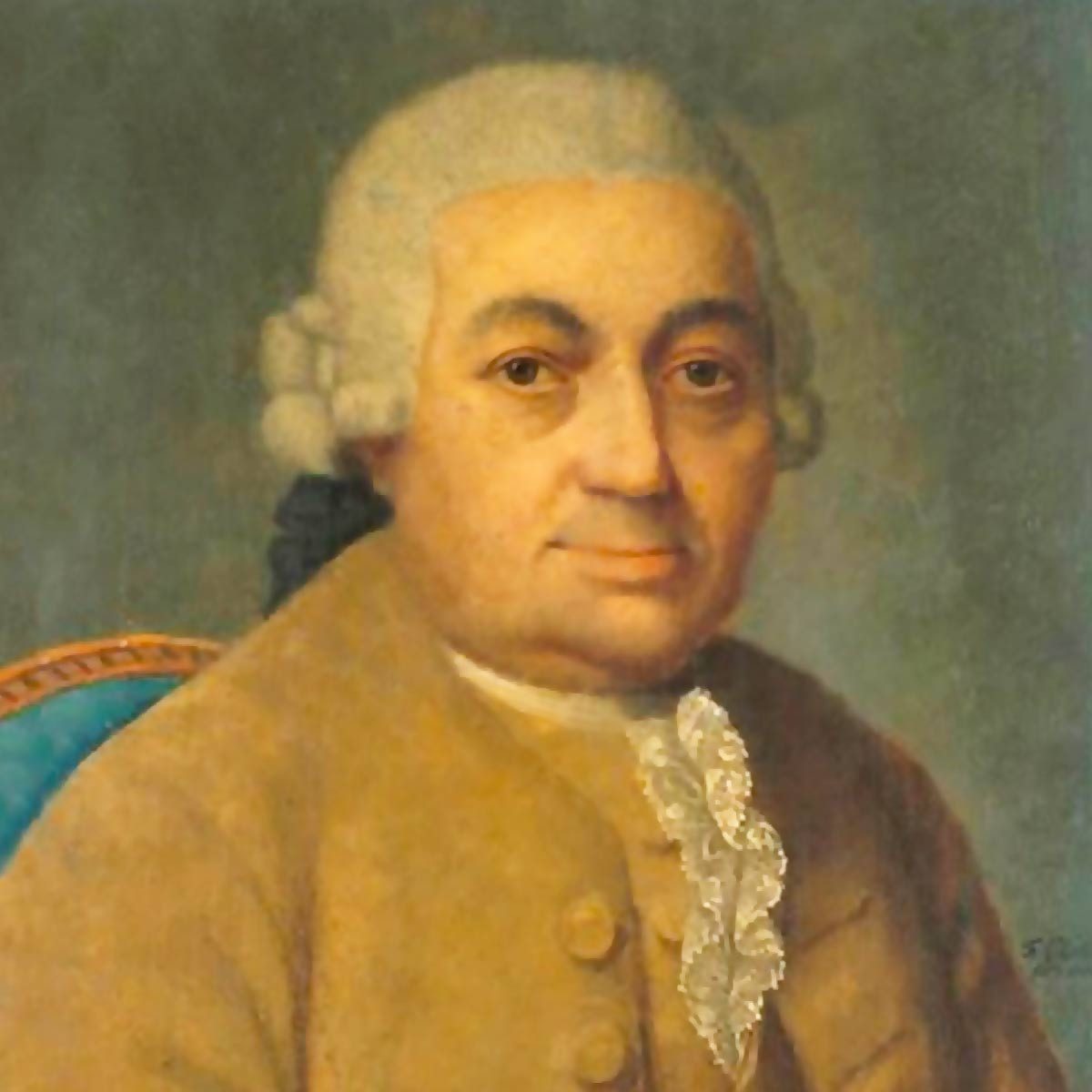# Carl Philipp Emanuel Bach

1 Music Sheets

• ###### Birth Name:

Carl Philipp Emanuel Bach

• ###### Born:

8th March 1714

• ###### Genres:

Carl Philipp Emanuel Bach, also formerly spelled Karl Philipp Emmanuel Bach, and commonly abbreviated C. P. E. Bach, was a German Classical period musician and composer, the fifth child and second surviving son of Johann Sebastian Bach and Maria Barbara Bach. His second name was given in honor of his godfather Georg Philipp Telemann, a friend of Johann Sebastian Bach. C. P. E. Bach was an influential composer working at a time of transition between his father's Baroque style and the Classical style that followed it. His personal approach, an expressive and often turbulent one known as empfindsamer Stil or 'sensitive style', applied the principles of rhetoric and drama to musical structures. Bach's dynamism stands in deliberate contrast to the more mannered galant style also then in vogue.Credit: Wikipedia

## Artist's Music Sheets

•( 8 ( w t Y y t r w r y o i Y y Y t Y o s D d s d s a p o i Y y Y t Y o s D d s a o a d h g D d D s D h l Z z l z l k j h g D d D s o Y t l h D H q W t i O s D d P i y E J g d h ( w E Y o P d s p P p s p P p D s o p D s o p d s I p j s I p G s y p s p I y P 5 ^ 9 w E e w Q 9 Q e y t E e E w E y o P p o p o I u y t E e E w E y o P p o I y I p d s P p P o P d h J j h j h G f d s P p Pw5 o P d h d P o q4 h d a o a d h sY o h o sY o h o ay o h o ay o h o Dt8 s D h l h D s E^ l h D s D h l gO s l s gO s l s fo s l s fo s l s H 4 % 8 q W w q 0 8 0 w t E W w W q W t i O o i o i u y t E W w W q W t i O o i u t u o s P O o O i O s g H h g h g f d s P O o O g s O i s O i t O i t W i t W * H g f g h g f g 8 O i u i O i u i 7 z g h H h g D d 8D h l h qJ H h g Dw d5 D d Ys o h o Ys o h o ya o g o ya o g o Pt o f o Pt o f o pi D l D pi D l D OE i d i OE i d i Yo S J S Yo S J S WI Y s Y WI Y s Y QY s l s QY s l s wY s D h l h D s h D s o g5 d a i Y 8 ( w t Y y t r w r y o i Y y Y t Y o s D d s d s a p o i Y y Y t Y o s D d s a o a d h g D d D s D h l Z z k l
Level: 3
Easy